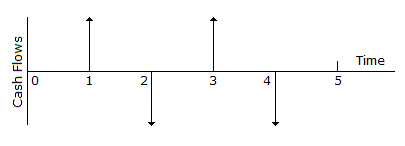# Civil Engineering - Engineering Economy

### Exercise :: Engineering Economy - Section 2

11.

A project construction cost estimate includes:

 A. the labour and material cost B. the equipment and over head cost C. the profit of the contractor D. All of these

Explanation:

No answer description available for this question. Let us discuss.

12.

In the cash flow diagram shown in the given figureA. The first disbursement occurs at the end of year 2 B. The second disbursement occurs at the end of year 4 C. The first receipt occurs at the end of year 1 D. The second receipt occurs at the end of year 3 E. All of these

Explanation:

No answer description available for this question. Let us discuss.

13.

Pick up the correct statement from the following:

 A. The receipts and disbursements in a given time interval are referred to as cash flow. B. The assumptions that all cash flows occur at the end of the interest period, is known as the end of period convention. C. A cash flow diagram is a graphical representation of cash flows drawn on a time scale. D. The cash flow diagram represents the statement of the problem and also includes what is given and what is to be found. E. All of the above.

Explanation:

No answer description available for this question. Let us discuss.

14.

The person desires to pay off the amount in 10 equal annual instalments. The amount of each instalment is :

 A. Rs 5638 B. Rs 6638 C. Rs 7738 D. None of these

Explanation:

No answer description available for this question. Let us discuss.

15.

Renu Bala deposits Rs 1200 now, Rs 800 two years from now and Rs 1000 five years from now. If the savings bank's rate of interest in 5%, she will receive an amount of Rs X 10 years from now, where X is

 A. Rs 3415 B. Rs 4225 C. Rs 4413 D. Rs 4826